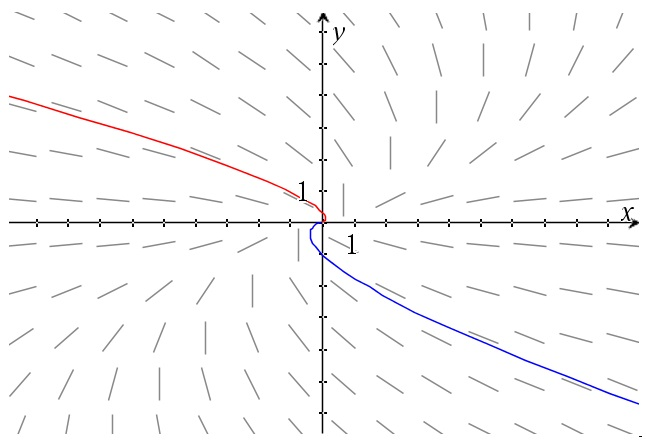# Homogenous differential equations – a more general type

Up a level : Differential Equations
Previous page : The order of operations
Next page : A harder example of solving a linear first order equationA homogenous differential equation is an equation of the form$y'f(x,y) + g(x,y) = 0$

where the two functions, f and g are both homogenous functions of the same degree.  These we can solve by doing the substitution$y = vx$

This means that$\frac{{dy}}{{dx}} = \frac{{d(vx)}}{{dx}} = \frac{{dv}}{{dx}}x + v\frac{{dx}}{{dx}} = \frac{{dv}}{{dx}}x + v$

or possibly even simpler, by just multiplying through by dx, or using the product rule directly$dy = xdv + vdx$

We could multiply our differential equation by dx to get$f(x,y)dy + g(x,y)dx = 0$

Doing the substitutions above gives us$f(x,vx)(xdv + vdx) + g(x,vx)dx = 0$

But since both our functions are homogenous of the same degree we get$f(x,vx) = {x^n}f(1,v),\quad g(x,vx) = {x^n}g(1,v)$

where p and q are constants. This gives us${x^n}f(1,v)(xdv + vdx) + {x^n}g(1,v)dx = 0$

or${x^n}f(1,v)xdv + {x^n}f(1,v)vdx + {x^n}g(1,v)dx = 0$

and thus${x^n}f(1,v)xdv + {x^n}f(1,v)vdx + {x^n}g(1,v)dx = 0$

or${x^n}(f(1,v)xdv + (f(1,v)v + g(1,v))dx) = 0$

By the null factor law we have$- f(1,v)xdv = (f(1,v)v + g(1,v))dx$

Separating the variables we get$- \frac{{f(1,v)}}{{f(1,v)v + g(1,v)}}dv = \frac{{dx}}{x}$

So we have been able to separate the variables. Observe that you are not supposed to follow the above as a recipe, but as a proof of concept.

Example 1

Let us look at the example from the previous page$xyy' + {x^2} + {y^2} = 0$

We can rewrite this as$xydy + ({x^2} + {y^2})dx = 0$

Next we do the substitutions$\left| \begin{gathered} y = vx \hfill \\ dy = xdv + vdx \hfill \\ \end{gathered} \right.$

We get$xvx(xdv + vdx) + ({x^2} + {v^2}{x^2})dx = 0$

This gives us${x^2}(vxdv + {v^2}dx + dx + {v^2}dx) = 0$

or$vxdv + (2{v^2} + 1)dx = 0$

Separating the variables gives us$- \frac{{dv}}{{2{v^2} + 1}} = \frac{{dx}}{x}$

and then we are at the same separable differential equation as in the previous page.

Example 2$(y - x)y' + y = 0$

So both our homogenous functions are of degree 1.$(y - x)dy + ydx = 0$

Next we do the substitution$\left| \begin{gathered} y = vx \hfill \\ dy = xdv + vdx \hfill \\ \end{gathered} \right.$

This gives us$(vx - x)(xdv + vdx) + vxdx = 0$

or$v{x^2}dv + {v^2}xdx - {x^2}dv - vxdx + vxdx = 0$

this gives us$x(vxdv + {v^2}dx - xdv) = 0$

or$vxdv + {v^2}dx - xdv = 0$

Separating the variables we get$x(v - 1)dv + {v^2}dx = 0$

or$\frac{{1 - v}}{{{v^2}}}dv = \frac{{dx}}{x}$

This gives us$\left( {\frac{1}{{{v^2}}} - \frac{1}{v}} \right)dv = \frac{{dx}}{x}$

Integrating this we get$- \frac{1}{v} - \ln |v| = \ln |x| + {C_1}$

or$- \frac{1}{v} + \ln |{v^{ - 1}}| = \ln |x| + {C_1}$

Exponentiation (e to the power of) of both sides gives us$\frac{{{e^{ - \frac{1}{v}}}}}{v} = Cx$

or$x\frac{{{e^{ - \frac{x}{y}}}}}{y} = Cx$

and finally${e^{ - \frac{x}{y}}} = Cy$

It is hard to make y your subject here (you need something called the Lambert W function), but we can make x your subject as in$x = - y\ln (Cy)$

Then you can reflect that curve trough y=x to get the inverse, and thus the curve of the wanted solution. This is what I have done in the graph below.Up a level : Differential Equations
Previous page : The order of operations
Next page : A harder example of solving a linear first order equationLast modified: Apr 1, 2019 @ 16:49